How Cheenta works to ensure student success?
Explore the Back-Story

# NMTC 2018 Stage II - KAPREKAR (Class 7, 8) - Problems and Solutions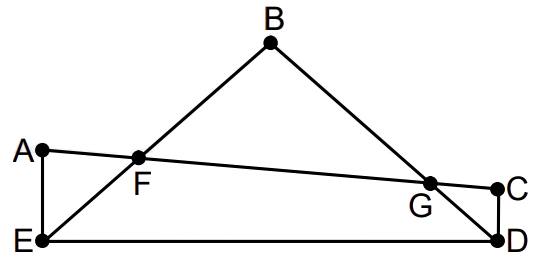###### Problem 1

A lucky year is one in which at least one date, when written in the form day / month / year, has the following property. The product of the month times the day equals the last two digits of the year. For example,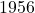is a lucky year because it has the date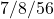where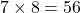, but 1962 is not a lucky year as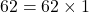or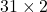, where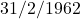is not a valid date. From 1900 to 2018 how many years are not lucky (not including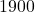and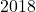) ? Give proper explanation for your answer.

###### Problem 2

In the figure given,andare right angles. If and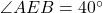and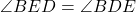, then find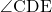.

###### Problem 3

(a)is a hexagon in which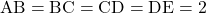and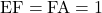. Its interior angle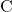is betweenand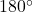and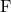is greater than. The rest of the angles areeach. What is its area?
(b) A convex polygon with '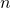' sides has all angles equal to, except one angle. List all possible values of.

###### Problem 4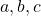are distinct non-zero reals such that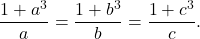Find all possible values of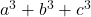###### Problem 5

Find the smallest positive integer such that it has exactly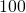different positive integer divisors includingand the number itself.

###### Problem 6

(a) What is the sum of the digits of the smallest positive integer which is divisible by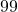and has all of its digits equal to?
(b) When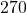is divided by the odd number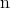, the quotient is a prime number and the remainder is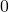. What is?

###### Problem 7

Consider the sums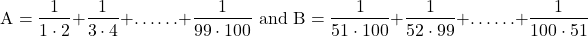Express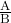as an irreducible fraction.

###### Problem 8

Letbe real numbers, not all of them are equal. Prove that if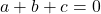, then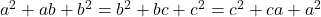.

Prove the converse, if, then.

###### Problem 1

A lucky year is one in which at least one date, when written in the form day / month / year, has the following property. The product of the month times the day equals the last two digits of the year. For example,is a lucky year because it has the datewhere, but 1962 is not a lucky year asor, whereis not a valid date. From 1900 to 2018 how many years are not lucky (not includingand) ? Give proper explanation for your answer.

###### Problem 2

In the figure given,andare right angles. If andand, then find.

###### Problem 3

(a)is a hexagon in whichand. Its interior angleis betweenandandis greater than. The rest of the angles areeach. What is its area?
(b) A convex polygon with '' sides has all angles equal to, except one angle. List all possible values of.

###### Problem 4are distinct non-zero reals such thatFind all possible values of###### Problem 5

Find the smallest positive integer such that it has exactlydifferent positive integer divisors includingand the number itself.

###### Problem 6

(a) What is the sum of the digits of the smallest positive integer which is divisible byand has all of its digits equal to?
(b) Whenis divided by the odd number, the quotient is a prime number and the remainder is. What is?

###### Problem 7

Consider the sumsExpressas an irreducible fraction.

###### Problem 8

Letbe real numbers, not all of them are equal. Prove that if, then.

Prove the converse, if, then.

This site uses Akismet to reduce spam. Learn how your comment data is processed.

### Knowledge Partner